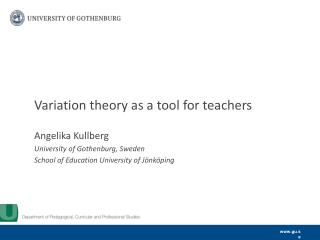Download PresentationVariation theory as a tool for teachers

# Variation theory as a tool for teachers - PowerPoint PPT PresentationDownload Presentation## Variation theory as a tool for teachers

- - - - - - - - - - - - - - - - - - - - - - - - - - - E N D - - - - - - - - - - - - - - - - - - - - - - - - - - -
##### Presentation Transcript

1. Variation theory as a tool for teachers Angelika Kullberg University of Gothenburg, Sweden School of Education University of Jönköping

2. Individual interviews with teachers about the planned lesson Individually planned and enacted lesson (video recorded) Learning study 1 Background: Research projectaboutif and howteacherschangetheir teaching afterparticipating in learning studies Learning study 2 Learning study 3 LGK- project: 4 studies in total (2 in Mathematics and 2 in Science) Project leader: Prof. Ulla Runesson, University of Jönköping Individual interviews with teachers about the planned lesson Individually planned and enacted lesson (video recorded) Stimulated recall interviews with individual teachers Angelika.Kullberg@gu.se

3. Variation theory (somebasicideas) Marton & Booth, 1997; Marton, Runesson & Tsui, 2004 • Learning is seen as the discernmentofaspectsthatyouhave not previouslydiscerned (cf. Gibson & Gibson, 1955). • “To discern an aspect, the learner must experience potential alternatives, that is, variation in a dimension corresponding to that aspect, against the background of invariance in other aspects of the same object of learning” (Marton & Pang, 2006, p. 193). • Therearenecessaryconditions for learning – criticalaspectsto be discerned. A criticalaspect is not the same as students’ difficulties, sincea criticalaspectsayswhat the students needtodiscern.

4. Lesson before the learning studies 1. Discussion about a task: “A person spent one third of his savings on a cd-player, and bought 3 cd for 165 cr. He paid 1975 cr. How much was his savings?” [The task is not solved] 2. Discussion in pairs about “what is an equation?” 3. Discussion about the meaning of the equal sign. E.g. 12·2=3·2·2·2was compared with 3·6≠18·2=36 4. The equation 3x+5=20 is solved with a visual representation. 5. The equations 3x+20=5 and 2x+3=3x+4 is discussed and found unsolvable for positive integers. 6. + 495=1975 is solved. (task 1) 7. A task about 3 persons ages, 3x+(x+5)+x=40 is discussed and solved. 8. Students work in pairs with making equations to given answers. Angelika.Kullberg@gu.se

5. “6·4” invariant, answersvary (meaningofequalsign) 1.Discussion about the equal sign. Example: calculate the area of a triangle. 6·4≠ =12 6·4 = =24 6·4= 12·2 = 24 Lesson after the learning studies ”4·” invariant,answersvary 2. Multiplication with x, comparing 3+3+3+3 = 4·3 (is not 43) with 4x= x+x+x+x=4·x (is 4x). operation invariant,examples vary 3. Discussion about = = 3, = = 5, = 4, = x 4. Discussion about addition and subtraction. 3+4=72+3+4=7+2 2+3+4-5=7+2-5 Discussion about multiplication, comparing 2·3+4=7·2with 2·3+4·2=7·2 ”3+4=7” invariant,operations vary Angelika.Kullberg@gu.se

6. Introducingx in an equationwithoneunknown ”3+4=7” invariant, ”3” varies, becomesx Angelika.Kullberg@gu.se

7. Introducing 2x and x in a division ”3+4=7” invariant, ”4” varies, becomes a part ofx ”3+4=7” invariant, ”3” varies, becomes a part ofx Angelika.Kullberg@gu.se

8. Conclusions Changes in teaching (video data) The teacheruses variation theoryas a toolto make aspectsofequationswithoneunknow explicit • In lesson 2 the teacher makes moredistinctions/contrasts. E.g. Between addition and multiplication (operations). • In lesson 2 the teacherbringsupcriticalaspectsofequations (interview data). • In lesson 2 the teacheruses ”variation and invariance” in a systematic way. E.g. 3+4=7 is kept invariant. • In lesson 2 the teacheroftenuseseveralexamples for the ”same idea” Angelika.Kullberg@gu.se

9. Lesson 2 The teacher: “…these [items] are more explicit in relation to the purpose. And I know that I can’t vary too much, because then I loose what I want to bring out. Because then students can get stuck at so many other things, they can see so many other things that I don’t want them to see at this moment, because that is not what they are supposed to learn this time” Angelika.Kullberg@gu.se